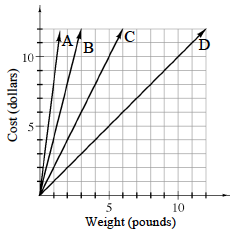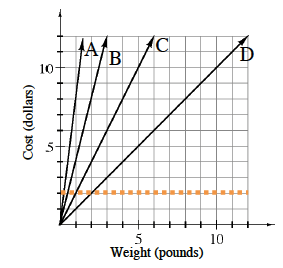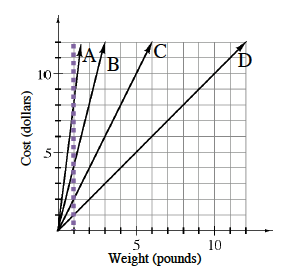### Home > MC2 > Chapter 7 > Lesson 7.1.3 > Problem7-29

7-29.

The graph at right shows the cost per pound of strawberries at four different stores.

1. At which store are strawberries about $2$ per pound?

2. What is the rate of cost of strawberries at store B?

3. Which store has the most expensive strawberries? How can you tell?Look at the $y$-axis and find the place where a cost of $2$ is represented.
Which line shows a store where $1$ pound of strawberries could be purchased for this price?

Look at the orange line on the graph for more help.

How many dollars does one pound of strawberries cost?

Look at the purple line on the graph for more help.

$4$ per poundCompare the cost of one pound of strawberries at each store.
Which store has the steepest line?